# Connes-Moscovici index theorem

(diff) ← Older revision | Latest revision (diff) | Newer revision → (diff)

Gamma index theorem,index theorem

A theorem [a3] which computes the pairing of a cyclic cocycle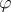of the group algebra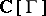with the algebraic-theory index of an invariant (pseudo-) differential operator on a coveringwith Galois group (or group of deck transformations)(cf. also Cohomology).

The ingredients of this theorem are stated in more detail below. Letbe a smooth compact manifold.

First, any-invariant, elliptic partial differential operator (cf. Elliptic partial differential equation)onhas an algebraic-theory index. The definition of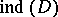is obtained using the boundary mapping on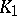applied to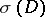, the principal symbol of(cf. also Symbol of an operator). This gives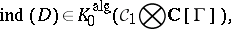whereis the algebra of trace-class operators on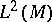(cf. also Trace). More generally, one can assume thatis an invariant pseudo-differential operator on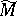(with nice support).

Secondly, it is known [a2] that any group-cohomology-cocycle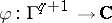ofcan be represented by an anti-symmetric function, and hence it defines a cyclic cocycle on the group algebraof the group. Moreover, the class of this cocycle in the periodic cyclic cohomology group, also denoted by, depends only on the class ofin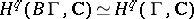. Here, as customary,denotes the classifying space of, whose simplicial cohomology is known to be isomorphic to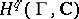, the group cohomology of.

Finally, any element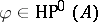gives rise to a group morphism, see [a2]. In particular, any group cocyclegives rise to a mapping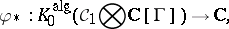using also the trace on.

The Connes–Moscovici index theorem now states [a3]): Let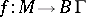be the mapping classifying the covering, let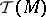be the Todd class of, and letbe the Chern character of the element in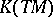defined by, as in the Atiyah–Singer index theorem (see [a1] and Index formulas). Then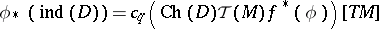is a pairing of a compactly supported cohomology class with the fundamental class of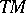. Here,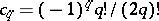.

The Connes–Moscovici index theorem is sometimes called the higher index theorem for coverings and is the prototype of a higher index theorem.

How to Cite This Entry:
Connes-Moscovici index theorem. Encyclopedia of Mathematics. URL: http://encyclopediaofmath.org/index.php?title=Connes-Moscovici_index_theorem&oldid=13937
This article was adapted from an original article by V. Nistor (originator), which appeared in Encyclopedia of Mathematics - ISBN 1402006098. See original article